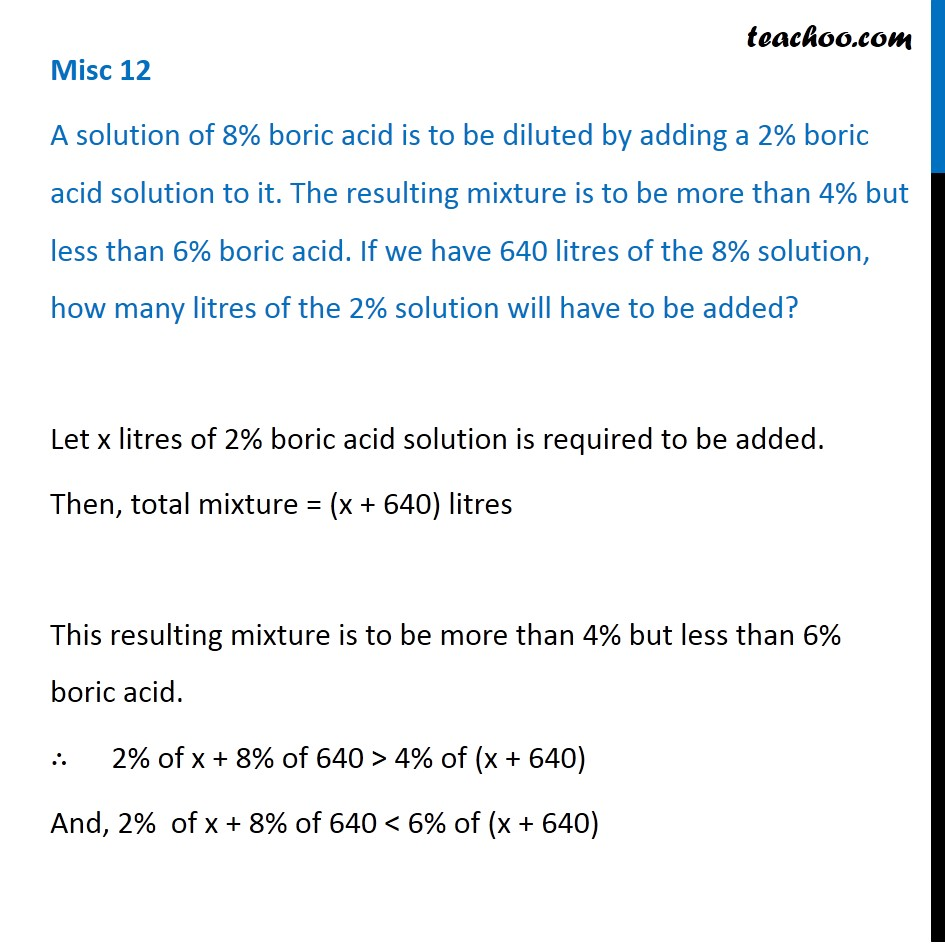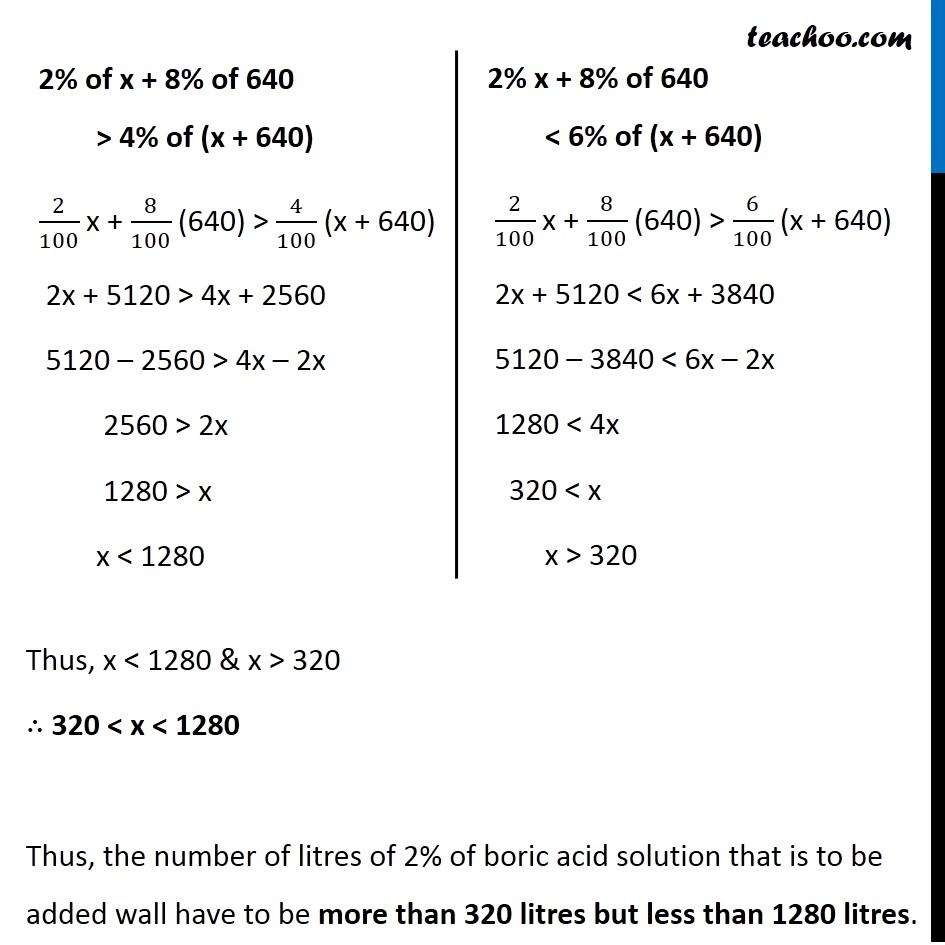Miscellaneous

Chapter 5 Class 11 Linear Inequalities
Serial order wiseLearn in your speed, with individual attention - Teachoo Maths 1-on-1 Class

### Transcript

Misc 12 A solution of 8% boric acid is to be diluted by adding a 2% boric acid solution to it. The resulting mixture is to be more than 4% but less than 6% boric acid. If we have 640 litres of the 8% solution, how many litres of the 2% solution will have to be added? Let x litres of 2% boric acid solution is required to be added. Then, total mixture = (x + 640) litres This resulting mixture is to be more than 4% but less than 6% boric acid. ∴ 2% of x + 8% of 640 > 4% of (x + 640) And, 2% of x + 8% of 640 < 6% of (x + 640) 2% of x + 8% of 640 > 4% of (x + 640) 2/100 x + 8/100 (640) > 4/100 (x + 640) 2x + 5120 > 4x + 2560 5120 – 2560 > 4x – 2x 2560 > 2x 1280 > x x < 1280 2% x + 8% of 640 < 6% of (x + 640) 2/100 x + 8/100 (640) > 6/100 (x + 640) 2x + 5120 < 6x + 3840 5120 – 3840 < 6x – 2x 1280 < 4x 320 < x x > 320 Thus, x < 1280 & x > 320 ∴ 320 < x < 1280 Thus, the number of litres of 2% of boric acid solution that is to be added wall have to be more than 320 litres but less than 1280 litres.